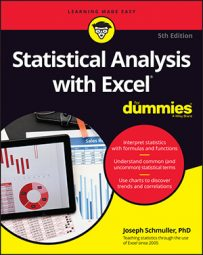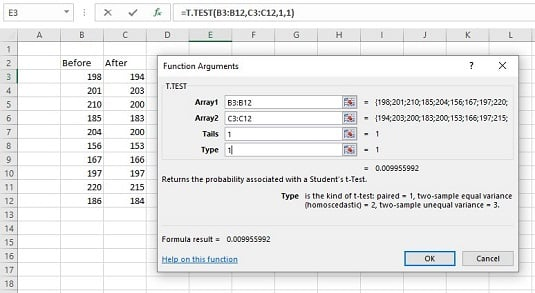##### Statistical Analysis with Excel For DummiesYou can use the worksheet function `T.TEST` for matched samples. Here is the Function Arguments dialog box for `T.TEST` along with data from a weight-loss example.The Function Arguments dialog box for T.TEST along with matched sample data.

Here are the steps to follow:

1. Enter the data for each sample into a separate data array and select a cell. For this example, the data for the Before sample are in column B and the data for the After sample are in column C.
2. From the Statistical Functions menu, select `T.TEST` to open the Function Arguments dialog box for `T.TEST`.
3. In the Function Arguments dialog box, enter the appropriate values for the arguments. In the Array1 box, type the sequence of cells that holds the data for one of the samples. In this example, the Before data are in B3:B12. In the Array2 box, type the sequence of cells that holds the data for the other sample. The After data are in C3:C12. The Tails box indicates whether this is a one-tailed test or a two-tailed test. In this example, it's a one-tailed test, so you type 1 in the Tails box. The Type box holds a number that indicates the type of t-test to perform. The choices are 1 for a paired test, 2 for two samples assuming equal variances, and 3 for two samples assuming unequal variances. Type 1. With values supplied for all the arguments, the dialog box shows the probability associated with the t value for the data. It does not show the value of t.
4. Click OK to put the answer in the selected cell.
The value in the dialog box is less than .05, so the decision is to reject H0.

If you assign the column headers as names for the respective arrays, the formula in the Formula bar can be

`=T.TEST(Before,After,1,1)`

That format might be easier to explain if you had to show the worksheet to someone.